# High School Physics : Electric Circuits

## Example Questions

### Example Question #1 : Understanding Electricity

A current of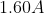flow in a wire.  How many electrons are flowing past any point in the wire per second?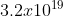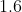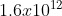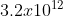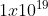Explanation:

The current is a measure of the amount of charge that passes a given point in a certain amount of time.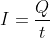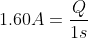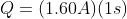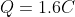To determine how many electrons are passing this point we need to look at the charge of 1 electron and do a conversion.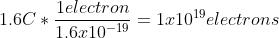### Example Question #1 : Electricity

The direction of conventional current is taken to be the direction that __________________ .

negative charges would flow

positive charges would flow

positive charges would flow

Explanation:

We often think of current flowing from the top of the battery to the bottom of the battery.  The top of the battery has a higher electrical potential than the bottom of the battery and is associated as being positive.  Charges interact in such a way where like charges repel and opposite charges attract.  Since we think of the charges traveling away from the positive end of the battery and toward the negative end of the battery, this would model the motion of a positive charge (away from positive and toward negative).  Since it is not protons that move through the circuit, but rather electrons.  It is more accurate to describe the flow of electrons from the negative side of the battery to the positive side.

### Example Question #61 : Electric Circuits

Why might a circuit breaker open if you plug too many electrical devices into a single circuit?

A circuit breaker will not “trip” no matter how many electrical devices you plug into the circuit

The resistance becomes too high

The current becomes too high

The voltage becomes too high

The current becomes too high

Explanation:

When plugin objects into a single circuit, these objects are connected in parallel as each one will receive the 120V from the electrical outlet. However, as additional objects are added, the current is thereby increased. Circuit breakers are designed to trip once the current reaches a maximum load and shuts down the circuit to protect the wires, and the devices that are plugged into the circuit as high current can damage these devices.

### Example Question #2 : Understanding Electricity

A 4.5V battery is connected to a bulb whose resistance is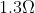. How many electrons leave the battery per minute?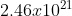electrons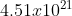electrons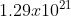electrons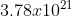electronselectrons

Explanation:

We first need to determine the current coming through the bulb. We can use Ohm’s law to determine this.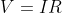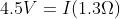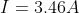Current by definition is the amount of charge per unit time.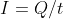We are looking at the number of electrons in one minute which is 60 seconds.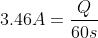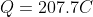We now know the amount of charge passing through in 60 seconds.  We know that the charge of the electron is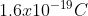.  We can use this to figure out how many electrons are going through.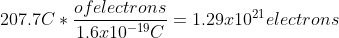### Example Question #3 : Understanding Electricity

Nothing happens when birds land on a power line, yet we are warned not to touch a power line with a ladder. What is the difference?

Most birds don’t understand the situation

Birds have extremely high internal resistance compared to humans

Dangerous current comes from the ground only

There is little to no voltage drop between a bird's two feet, but there is a significant voltage drop between the top of a ladder touching a power line and the bottom of the ladder on the ground

There is little to no voltage drop between a bird's two feet, but there is a significant voltage drop between the top of a ladder touching a power line and the bottom of the ladder on the ground

Explanation:

Electricity travels from the point of highest potential to the lowest potential. We measure the difference between these two potentials as the voltage. Since both of the birds' feet are on the wire, both feet have the same potential. However, if the ladder touches the power line and the bottom of the ladder is on the ground, there is a much higher potential difference (as the ground is at 0 potential).  Therefore the electricity will travel down the ladder!

### Example Question #4 : Understanding Electricity

When a light switch is turned on, the light comes on immediately because

The lightbulb may be old with low resistance. It would take longer if the bulb were new and had high resistance

The electrons coming from the power source move through the initially empty wires very fast

The electrons already in the wire are instantly “pushed” by the voltage difference

The electricity bill is paid. The electric company can make it take longer when the bill is unpaid

The electrons already in the wire are instantly “pushed” by the voltage difference

Explanation:

It's kind of like when you turn on the faucet; the water doesn't have to travel from the water tower to your house before it starts flowing. Similarly, the electrons are always in the circuit. Once the switch is turned on, there is a voltage difference present, which is what then pushes these electrons through the circuit (like turning on the faucet).

### Example Question #1 : Resistivity

Ten resistors, each with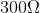resistance, are set up in series. What is their equivalent resistance?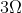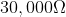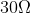Explanation:

For resistors aligned in series, the equivalent resistance is the sum of the individual resistances.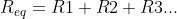Since all the resistors in this problem are equal, we can simplify with multiplication.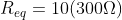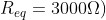### Example Question #61 : Electric Circuits

What is the total resistance of a parallel circuit with resistors of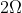,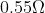, and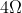?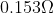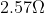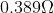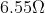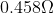Explanation:

The formula for resistance in parallel is: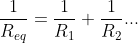We are given the values for each individual resistor, allowing us to solve for the total resistance.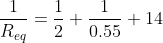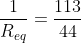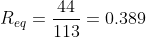### Example Question #951 : High School Physics

An electric circuit is set up in series with five resistors. If the resistors remain the same, but the circuit is now set up with the resistors in parallel, how would this affect the total resistance?

Resistance would decrease substantially

We need to know the numerical values to solve

Resistance would remain unchanged

Resistance would increase substantially

Resistance would decrease substantially

Explanation:

Think of resistors as doors, preventing the flow of people (electrons). Imagine the following scenarios: a large group of people are in a room and all try to leave at once. If the five resistors are in series, that's like having all of these people trying to go through all five doors before they can leave. In a circuit, all the electrons in the current mast pass through every resistor.

If the resistors are in parallel, it's like having five separate doors from the room. All of a sudden, the group can leave MUCH faster, encountering less resistance to their flow out of the room. The path of the electrons can split, allowing each particle to pass through only one resistor.

From a formula perspective, the resistors in series are simply summed together to find the equivalent resistance.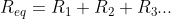In parallel, however, the reciprocals are summed to find the reciprocal equivalent resistance.Adding whole numbers will always give you a much greater result than adding fractions. For the exact same set of resistors, arrangement in series will have a greater total resistance than arrangement in parallel.

### Example Question #6 : Resistivity

When current in a circuit crosses a resistor, energy is lost. What form does this lost energy most commonly take?

The energy is converted into sound

The energy is converted into heat

The energy is converted into light

The energy is not converted; it simply disappears

The energy is converted into motion